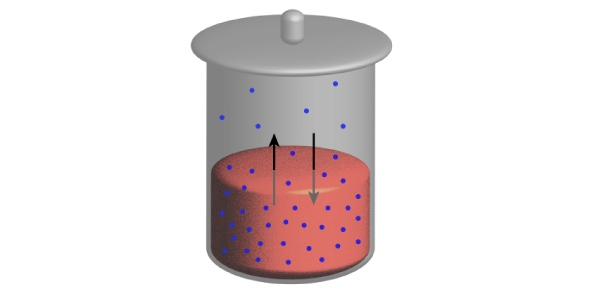What is the vapour pressure of an aqueous solution of sucrose with - ProProfs Discuss+ Ask Question

# What is the vapour pressure of an aqueous solution of sucrose with mole fraction 0.2?Change Image    Delete

Asked by Ejazmoaz, Last updated: Aug 04, 2021

###Request 0FollowShareAnswer AnonymouslyAnswer LaterCopy LinkJ.Spencer, Knowledge enthusiast, Tokyo

The answer is A.19.04 mm Hg.

According toRaoult's law, the vapor pressure (p) in a solution with a non-volatile solute is equal to the product of mole fraction of solvent (x1) and vapor pressure of the solvent (p1).

Therefore,

p= p1*x1

Now,

Given:

Vapour pressure of Water (p1): 23.8mmHg

Mole fraction of sugar (x2) = 0.2

Hence,

Mole Fraction of Water (x1) = 1-x2 = 1-0.2= 0.8.

Thus,

p = p1*x1

p= 23.8*0.8

p= 19.04

Hence, the vapor pressure of the aqueous solution is 19.04 mm Hg.John Smith19.04 mm HgSearch for Google imagesSelect a recommended image
Upload from your computerCancelSearch for Google imagesSelect a recommended image
Upload from your computerCancelSearch for Google imagesSelect a recommended image
Upload from your computerCancel